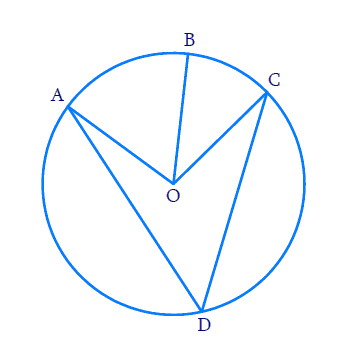# Ex.10.5 Q1 Circles Solution - NCERT Maths Class 9

Go back to  'Ex.10.5'

## Question

In the given figure, $$A,\, B$$ and $$C$$ are three points on a circle with center $$O$$ such that \begin{align}\angle {BOC}=30^{\circ} \text { and } \angle {AOB}=60^{\circ} \end {align}If  $$D$$ is a point on the circle other than the arc $$ABC,$$  find \begin{align} \angle {ADC} \end{align}Video Solution
Circles
Ex 10.5 | Question 1

## Text Solution

What is known?

Two angles subtended by arcs at the centre.

What is unknown?

Value of  $$\angle {ADC.}$$

Reasoning:

The angle subtended by an arc at the centre is double the angle subtended by it at any point on the remaining part of the circle.

Steps:

\begin{align} \angle {AOC} &=\angle {AOB}+\angle {BOC} \\ &=90^\circ \end{align}

By Theorem $$10.8$$ ,

\begin{align} \angle {AOC} &=2 \angle {ADC} \\ \angle ADC &= \frac{1}{2}\angle AOC\\\angle ADC &= \frac{1}{2} \times 90 = \,45^\circ \\\therefore \angle ADC &= \,45^\circ \end{align}

Video Solution
Circles
Ex 10.5 | Question 1

Learn from the best math teachers and top your exams

• Live one on one classroom and doubt clearing
• Practice worksheets in and after class for conceptual clarity
• Personalized curriculum to keep up with school